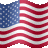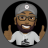# Projection direction of the next 3 bars

L

#### lents

##### New member
I am trying to conceptualize a time series Model in the script and cannot get my head around how to plot future calculations based on the past data (outside of the current bar). I am trying to forecast 2 (or more) periods ahead from the most current based on the parameters of past data. In the code below, I found some indication about the future offset, however I need to have a recurring calculation that runs ahead using the values from the past. For example I am looking at back values of periods back of 1 , 2 3 4 12 and 13 to calculate the next 2 values, however I am having a difficulty to project the calculation as a one more observation based on the most recent calculated value. It seems that the code is offsetting the indicator forward instead of using the last calculated value

Could you please help or point me in the right direction on how to have the projection be next after the last bar/ value, and then make that projection as part of the past data and continue cycling through forward? I understand that the below code is a spagetti code, but I appreciate your looking into it. Thank you in advance for your help/guidance

Code:
``````declare lower;
input symbol5 = "SPY";
input price = FundamentalType.CLOSE;
input open = FundamentalType.OPEN;
input low = low;
input dif_length_1 = 1;
input lineLength = 1;

input    AR1    =    1    ;
input    AR2    =   2    ;
input    AR3    =   3    ;
input    AR4    =   4    ;
input    AR5    =   5    ;
input    AR6    =   6    ;
input    AR7    =   7    ;
input    AR8    =   8    ;
input    AR9    =   9    ;
input    AR10    =   10    ;
input    AR11    =  11    ;
input    AR12    =  12    ;
input    AR13    =  13    ;

#this step is setting my base ( a perentage calculation)
def pct_Dif_1 = ((Fundamental(price, symbol5)) - (Fundamental( price, symbol5)[dif_length_1])) / (Fundamental( price, symbol5)[dif_length_1]);

# this step is calculating the derivative  forecasted base from previous step
def fcst_SPY_AR = ((pct_Dif_1 * 0.19454 - pct_Dif_1[AR1] * 1.11259 - pct_Dif_1[AR2] * 0.026559 - pct_Dif_1[AR3] * 0.56788 - pct_Dif_1[AR11] * 0.11252 - pct_Dif_1[AR12] * 0.13252));

def resid_err =  pct_Dif_1 - fcst_SPY_AR;

def fcst_resid_err = (.65692 * resid_err + 0.16472 * resid_err[AR1] - 0.09965 * resid_err[AR2]);

def fcst_spy_1 = ((pct_Dif_1 * 0.19454 - pct_Dif_1[AR1] * 1.11259 - pct_Dif_1[AR2] * 0.026559 - pct_Dif_1[AR3] * 0.56788 - pct_Dif_1[AR11] * 0.11252 - pct_Dif_1[AR12] * 0.13252)) - 0.00043 + fcst_resid_err ;

def resid_err2 =  pct_Dif_1 - fcst_spy_1;
def fcst_resid_err2 = (.65692 * resid_err2 + 0.16472 * resid_err2[AR1] - 0.09965 * resid_err2[AR2]);

#this step is is looking for the last value calculated by the derivative base
def lastBar_SPY = !IsNaN(fcst_spy_1) && IsNaN(fcst_spy_1[-1]);
def lastClose_SPY = if lastBar_SPY then fcst_spy_1 else lastClose_SPY  ;

#based on the last calculated  value I want to calculate the plus one outside using the last fcst_spy_1 value. Note that I am attempting to move forward by changing the numbers next to the lastClose_SPY
def fcst_spy_2 = ((lastClose_SPY[-1] * 0.19454 - pct_Dif_1[AR1] * 1.11259 - pct_Dif_1[AR2] * 0.026559 - pct_Dif_1[AR3] * 0.56788 - pct_Dif_1[AR11] * 0.11252 - pct_Dif_1[AR12] * 0.13252)) - 0.00043 + fcst_resid_err2 ;

# this step identifies the last value  from the previous calculation and adds one more forecasted value
def lastBar_SPY2 = !IsNaN( fcst_spy_2) && IsNaN( fcst_spy_2[-1]);
def lastClose_SPY2 = if lastBar_SPY2 then fcst_spy_2 else fcst_spy_2   ;

def fcst_spy_3 = ((lastClose_SPY2 * 0.19454 - lastClose_SPY2  * 1.11259 - pct_Dif_1[AR1] * 0.026559 - pct_Dif_1[AR2] * 0.56788 - pct_Dif_1[AR10] * 0.11252 - pct_Dif_1[AR11] * 0.13252)) - 0.00043 + fcst_resid_err ;

def lastBar_SPY3 = !IsNaN( fcst_spy_3) && IsNaN( fcst_spy_3[-1]);
plot lastClose_SPY3 = if lastBar_SPY3 then fcst_spy_3 else fcst_spy_3  ;
# the result gives me 2 forecasted periods calculated ahead, but not the right ones``````

Last edited by a moderator:Leavitt Projection Indicator for ThinkorSwim Indicators 10Time Series Projection Trend for ThinkorSwim Indicators 8Seeking Direction of MA ThinkScript Questions 0